Students those who are preparing for the 12th Physics exam can download this Samacheer Kalvi 12th Physics Book Solutions Questions and Answers for Chapter 7 Dual Nature of Radiation and Matter from here free of cost. These Tamilnadu State Board Solutions for Class 12th Physics PDF cover all Chapter 7 Dual Nature of Radiation and Matter Questions and Answers. All these concepts of Samacheer Kalvi 12th Physics Book Solutions Questions and Answers are explained very conceptually as per the board prescribed Syllabus & guidelines.

## Tamilnadu Samacheer Kalvi 12th Physics Solutions Chapter 7 Dual Nature of Radiation and Matter

### Samacheer Kalvi 12th Physics Dual Nature of Radiation and Matter Textual Evaluation Solved

Samacheer Kalvi 12th Physics Dual Nature of Radiation and Matter Multiple Choice Questions

Question 1.
The wavelength λe of an electron and λp of a photon of same energy E are related by …….. .
(a) λp ∝ λe
(b) λp ∝ $$\sqrt { { \lambda }_{ e } }$$
(c) λp ∝ $$\frac { 1 }{ \sqrt { { \lambda }_{ e } } }$$
(d) λp ∝ $${ \lambda }_{ e }^{ 2 }$$
(d) λp ∝ $${ \lambda }_{ e }^{ 2 }$$
Hint:
de broglie wavelength of electron, λe = $$\frac { h }{ \sqrt { 2mE } }$$
∴ ie λe ∝ $$\frac { 1 }{ \sqrt { E } }$$ ⇒ $${ \lambda }_{ e }^{ 2 }$$ ∝ $$\frac { 1 }{ E }$$ …… (1)
de-Broglie wavelength of proton
λp = $$\frac { hc }{ E }$$
λp ∝ $$\frac { 1 }{ E }$$ …… (2)
From (1) and (2)
$${ \lambda }_{ e }^{ 2 }$$ ∝ λp i.e., λp ∝ $${ \lambda }_{ e }^{ 2 }$$

Question 2.
In an electron microscope, the electrons are accelerated by a voltage of 14 kV. If the voltage is changed to 224 kV, then the de Broglie wavelength associated with the electrons would …….. .
(a) increase by 2 times
(b) decrease by 2 times
(c) decrease by 4 times
(d) increase by 4 times
(c) decrease by 4 times
Hint:
At Voltage, V = 14 kV
de-Broglie wavelength of electron,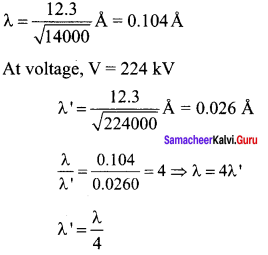de-Broglie wavelength of electron is decreased by 4 times

Question 3.
A particle of mass 3 x 10-6 g has the same wavelength as an electron moving with a velocity
6 x 106 ms-1 The velocity of the particle is …….. .
(a) 1.82 x 10-18 ms-1
(b) 9 x 10-2 ms-1
(c) 3 x 10-31 ms-1
(d)1.82 x 10-15 ms-1
(d)1.82 x 10-15 ms-1
Hint: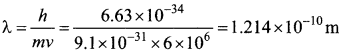Velocity of the particle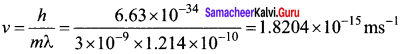Question 4.
When a metallic surface is illuminated with radiation of wavelength λ, the stopping potential is V. If the same surface is illuminated with radiation of wavelength 2λ, the stopping potential is $$\frac { V }{ 4 }$$. The threshold wavelength for the metallic surface is ………. .
(a) 4λ
(b) 5λ
(c) $$\frac { 5 }{ 2 }$$ λ
(d) 3λ
(d) 3λ
Hint: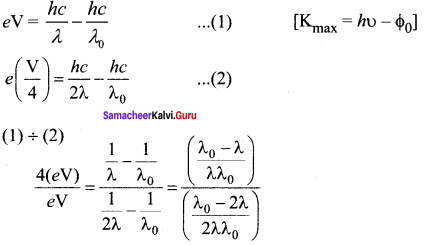On solving we get, λ0 = 3λ

Question 5.
If a light of wavelength 330 nm is incident on a metal with work function 3.55 eV, the electrons are emitted. Then the wavelength of the emitted electron is (Take h = 6.6 x 10-34 Js) ……… .
(a) < 2.75 x 10-9 m
(b) ≥ 2.75 x 10-9 m
(c) < 2.75 x 10-12um
(d) ≤ 2.75 x 10-10um
(a) < 2.75 x 10-9 m
Hint:
Maximum KE of emitted electron is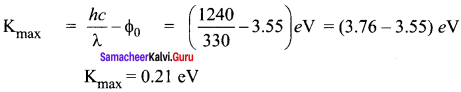de-Broglie wavelength of emitted electron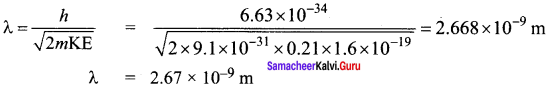The Two wavelength of the emitted electron is < 2.75 x 10-9 m

Question 6.
A photoelectric surface is illuminated successively by monochromatic light of wavelength λ and $$\frac { λ }{ 2 }$$ . If the maximum kinetic energy of the emitted photoelectrons in the second case is 3 times that in the first case, the work function at the surface of material is …….. .
(a) $$\frac { hc }{ λ }$$
(b) $$\frac { 2hc }{ λ }$$
(c) $$\frac { hc }{ 3λ }$$
(d) $$\frac { hc }{ 2λ }$$
(d) $$\frac { hc }{ 2λ }$$
Hint:
KE1 = $$\frac { hc }{ λ }$$ – Φ ……. (2)
3KE1 = $$\frac { 2hc }{ λ }$$ – Φ
KE1 = $$\frac { 2hc }{ 3λ }$$ – $$\frac { Φ }{ 3λ}$$ ….. (2)
Equating (1) and (2)
$$\frac { hc }{ λ }$$ – Φ = $$\frac { 2hc }{ 3λ }$$ – $$\frac { Φ }{ 3λ}$$
$$\frac { hc }{ 3λ }$$ = $$\frac { 2Φ }{ 3λ}$$ ⇒ Φ = $$\frac { hc }{ 2λ }$$

Question 7.
In photoelectric emission, a radiation whose frequency is 4 times threshold frequency of a certain metal is incident on the metal. Th en the maximum possible velocity of the emitted electron will be ……….. .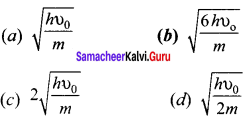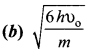Hint:
From Einstein’s photoelectric equation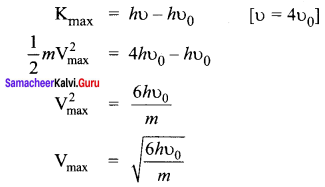Question 8.
Two radiations with photon energies 0.9 eV and 3.3 eV respectively are falling on a metallic surface successively. If the work function of the metal is 0.6 eV, then the ratio of maximum speeds of emitted electrons will be ………..
(a) 1 : 4
(b) 1 : 3
(c) 1 : 1
(d) 1 : 9
(b) 1 : 3
Hint: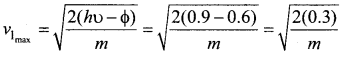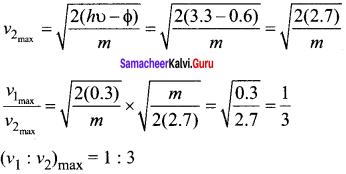Question 9.
A light source of wavelength 520 nm emits 1.04 x 1015 photons per second while the second source of 460 nm produces 1.38 x 1015 photons per second. Then the ratio of power of second source to that of first source is ……… .
(a) 1.00
(b) 1.02
(c) 1.5
(d) 0.98
(c) 1.5
Hint:
Power: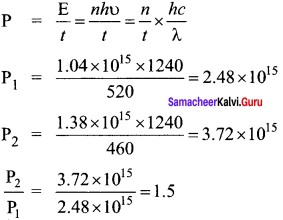Question 10.
The mean wavelength of light from sun is taken to be 550 nm and its mean power is 3.8 x 1026 W. The number of photons received by the human eye per second on the average from sunlight is of the order of ………. .
(a) 1045
(b) 1042
(c) 1054
(d) 1051
(a) 1045
Hint: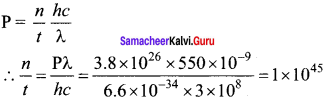Question 11.
The threshold wavelength for a metal surface whose photoelectric work function is 3.313 eV is
(a) 4125 Å
(b) 3750 Å
(c) 6000 Å
(d) 2062.5 Å
(b) 3750 Å
Hint: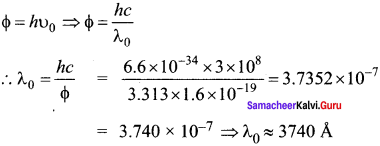Question 12.
A light of wavelength 500 nm is incident on a sensitive plate of photoelectric work function 1.235 eV. The kinetic energy of the photo electrons emitted is be (Take h = 6.6 x 10-34 Js)
(a) 0.58 eV
(b) 2.48 eV
(c) 1.24 eV
(d) 1.16 eV
(c) 1.24 eV
Hint: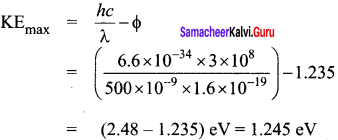Question 13.
Photons of wavelength λ are incident on a metal. The most energetic electrons ejected from the metal are bent into a circular arc of radius R by a perpendicular magnetic field having magnitude B. The work function of the metal is ……… .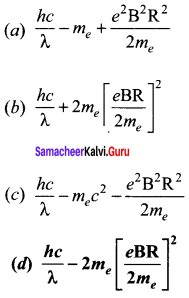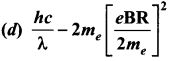Hint:
Magnetic lorentz force = Centripetal force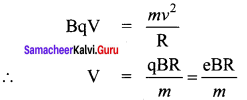From Einstein’s photo electric equation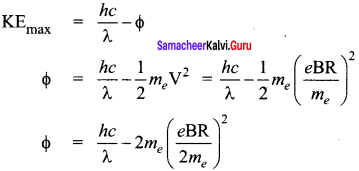Question 14.
The work functions for metals A, B and C are 1.92 eV, 2.0 eV and 5.0 eV respectively. The metals which will emit photoelectrons for a radiation of wavelength 4100 Å is/are ………. .
(a) A only
(b) both A and B
(c) all these metals
(d) none
(b) both A and B
Hint: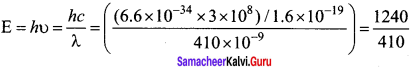E = 3.04 eV
Since energy of incident radiation is greater than the work function of metals A and B. So metal A and B will emit photoelectrons.

Question 15.
Emission of electrons by the absorption of heat energy is called ……… emission.
(a) photoelectric
(b) field
(c) thermionic
(d) secondary
(c) thermionic

Samacheer Kalvi 12th Physics Dual Nature of Radiation and Matter Short Answer Questions

Question 1.
Why do metals have a large number of free electrons?
In metals, the electrons in the outer most shells are loosely bound to the nucleus. Even at room temperature, there are a large number of free electrons which are moving inside the metal in a random manner.

Question 2.
Define work function of a metal. Give its unit.
The minimum energy needed for an electron to escape from the metal surface is called work function of that metal. It’s unit is electron volt (eV).

Question 3.
What is photoelectric effect?
The ejection of electrons from a metal plate when illuminated by light or any other electromagnetic radiation of suitable wavelength (or frequency) is called photoelectric effect.

Question 4.
How does photocurrent vary with the intensity of the incident light?
Photocurrent – the number of electrons emitted per second is directly proportional to the intensity of the incident light.

Question 5.
Give the definition of intensity of light and its unit.
Intensity of light refer to the strength or brightness or amount of light produced by a specific source. It’s unit is candela (cd)

Question 6.
How will you define threshold frequency?
For a given surface, the emission of photoelectrons takes place only if the frequency of incident light is greater than a certain minimum frequency called the threshold frequency.

Question 7.
What is a photo cell? Mention the different types of photocells.
photocells: Photo electric cell or photo cell is a device which converts light energy into electrical energy. It works on the principle of photo electric effect.
Types:

• Photo emissive cell
• Photo voltaic cell
• Photo conductive cell

Question 8.
Write the expression for the de Broglie wavelength associated with a charged particle of charge q and mass m, when it is accelerated through a potential V.
An electron of mass m is accelerated through a potential difference of V volt. The kinetic energy acquired by the electron is given by
$$\frac { 1 }{ 2 }$$ mv2 = eV
Therefore, the speed v of the electron is v = $$\sqrt { \frac { 2ev }{ m } }$$
Hence, the de Broglie wavelength of the electron is λ = $$\frac { h }{ mv }$$ = $$\frac { h }{ \sqrt { 2emV } }$$

Question 9.
State de Broglie hypothesis.
De Broglie hypothesis, all matter particles like electrons, protons, neutrons in motion are associated with waves.

Question 10.
Why we do not see the wave properties of a baseball?
Due to the large mass of a baseball, the de Broglie wavelength
[λ = $$\frac { h }{ mv }$$] associated with a moving baseball is very small. Hence its wave nature is not visible.

Question 11.
A proton and an electron have same kinetic energy. Which one has greater de Broglie wavelength. Justify.
de-Broglie wavelength of the particle is λ = $$\frac { h }{ p }$$ = $$\frac { h }{ \sqrt { 2mK } }$$
i.e. λ ∝ $$\frac { h }{ \sqrt { m } }$$
As me << mp, so λe >> λp
Hence protons have greater de-Broglie wavelength.

Question 12.
Write the relationship of de Broglie wavelength λ associated with a particle of mass m in terms of its kinetic energy K.
Kinetic energy of the particle, K = $$\frac { 1 }{ 2 }$$ mv2 = $$\frac { { P }^{ 2 } }{ 2m }$$
p = $$\sqrt { 2mK }$$
de-Broglie wavelength of the particle λ = $$\frac { h }{ p }$$ = $$\frac { h }{ \sqrt { 2mK } }$$

Question 13.
Name an experiment which shows wave nature of the electron. Which phenomenon was observed in this experiment using an electron beam?

• Davisson – Germer experiment confirmed the wave nature of electrons.
• They demonstrated that electron beams are diffracted when they fall on crystalline solids.

Question 14.
An electron and an alpha particle have same kinetic energy. How are the de Broglie wavelengths associated with them related?
[λ = $$\frac { h }{ p }$$]
Kinetic energy of the particle K = $$\frac { 1 }{ 2 }$$ mv2 = $$\frac { { P }^{ 2 } }{ 2m }$$ = $$\frac { { h }^{ 2 } }{ 2m{ \lambda }^{ 2 } }$$
i.e. λ = $$\frac { h }{ \sqrt { 2mK } }$$ ; λ ∝ $$\frac { 1 }{ \sqrt { m} }$$
$$\frac { { \lambda }_{ e } }{ { \lambda }_{ \alpha } }$$ = $$\sqrt { \frac { { m }_{ \alpha } }{ { m }_{ e } } }$$

Samacheer Kalvi 12th Physics Dual Nature of Radiation and Matter Long Answer Questions

Question 1.
What do you mean by electron emission? Explain briefly various methods of electron emission.
Electron emission:
1. Free electrons possess some kinetic energy and this energy is different for different electrons. The kinetic energy of the free electrons is not sufficient to overcome the surface barrier.

2. Whenever an additional energy is given to the free electrons, they will have sufficient energy to cross the surface barrier. And they escape from the metallic surface.

3. The liberation of electrons from any surface of a substance is called electron emission.

There are mainly four types of electron emission which are given below.
(i) Thermionic emission:
When a metal is heated to a high temperature, the free electrons on the surface of the metal get sufficient energy in the form of thermal energy so that they are emitted from the metallic surface. This type of emission is known as thermionic emission.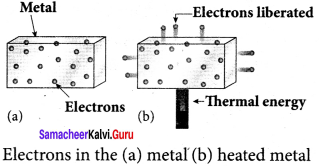The intensity of the thermionic emission (the number of electrons emitted) depends on the metal used and its temperature.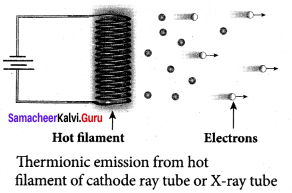Examples: cathode ray tubes, electron microscopes, X-ray tubes etc.

(ii) Field emission:
Electric field emission occurs when a very strong electric field is applied across the metal. This strong field pulls the free electrons and helps them to overcome the surface barrier of the metal.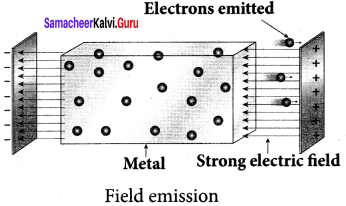Examples: Field emission scanning electron microscopes, Field-emission display etc.

(iii) Photo electric emission:
When an electromagnetic radiation of suitable frequency is incident on the surface of the metal, the energy is transferred from the radiation to the free electrons. Hence, the free electrons get sufficient energy to cross the surface barrier and the photo electric emission takes place. The number of electrons emitted depends on the intensity of the incident radiation.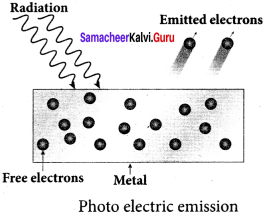Examples: Photo diodes, photo electric cells etc.

(iv) Secondary emission:
When a beam of fast moving electrons strikes the surface of the metal, the kinetic energy of the striking electrons is transferred to the free electrons on the metal surface. Thus the free electrons get sufficient kinetic energy so that the secondary emission of , electron occurs.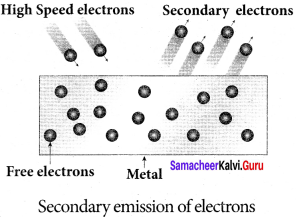Examples: Image intensifies, photo multiplier tubes etc.

Question 2.
Briefly discuss the observations of Hertz, Hallwachs and Lenard.
Hertz observation:
1. In 1887, Heinrich Hertz first became successful in generating and detecting electromagnetic wave with his high voltage induction coil to cause a spark discharge between two metallic spheres.

2. When a spark is formed, the charges will oscillate back and forth rapidly and the electromagnetic waves are produced.

3. The electromagnetic waves thus produced were detected by a detector that has a copper wire bent in the shape of a circle. Although the detection of waves is successful, there is a problem in observing the tiny spark produced in the detector.

4. In order to improve the visibility of the spark, Hertz made many attempts and finally noticed an important thing that small detector spark became more vigorous when it was exposed to ultraviolet light.

5. The reason for this behaviour of the spark was not known at that time. Later it was found that it is due to the photoelectric emission.

6. Whenever ultraviolet light is incident on the metallic sphere, the electrons on the outer surface are emitted which caused the spark to be more vigorous.

Hallwachs’ observation: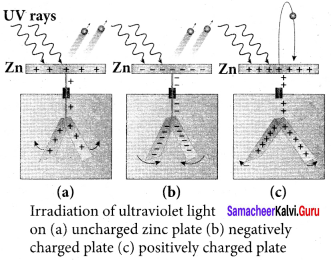8. In 1888, Wilhelm Hallwachs, a German physicist, confirmed that the strange behaviour of the spark is due to the action of ultraviolet light with his simple experiment.

9. A clean circular plate of zinc is mounted on an insulating stand and is attached to a gold leaf electroscope by a wire. When the uncharged zinc plate is irradiated by ultraviolet light from an arc lamp, it becomes positively charged and the leaves will open.

10. Further, if the negatively charged zinc plate is exposed to ultraviolet light, the leaves will close as the charges leaked away quickly. If the plate is positively charged, it becomes more positive upon UV rays irradiation and the leaves will open further.

11. From these observations, it was concluded that negatively charged electrons were emitted from the zinc plate under the action of ultraviolet light.

Lenard’s observation:
1. In 1902, Lenard studied this electron emission phenomenon in detail. The apparatus consists of two metallic plates A and C placed in an evacuated quartz bulb. The galvanometer G and battery B are connected in the circuit.

2. When ultraviolet light is incident on the negative plate C, an electric current flows in the circuit that is indicated by the deflection in the galvanometer. On other hand, if the positive plate is irradiated by the ultraviolet light, no current is observed in the circuit.

3. From these observations, it is concluded that when ultraviolet light falls on the negative plate, electrons are ejected from it which are attracted by the positive plate A. On reaching the positive plate through the evacuated bulb, the circuit is completed and the current flows in it.

4. Thus, the ultraviolet light falling on the negative plate causes the electron emission from the surface of the plate.

Question 3.
Explain the effect of potential difference on photoelectric current.
Effect of potential difference on photoelectric current:
1.  To study the effect of potential difference V between the electrodes on photoelectric current, the frequency and intensity of the incident light are kept constant. Initially the potential of A is kept positive with respect to C and the cathode is irradiated with the given light.

2. Now, the potential of A is increased and the corresponding photocurrent is noted. As the potential of A is increased, photocurrent is also increased. However a stage is reached where photocurrent reaches a saturation value (saturation current) at which all the photoelectrons from C are collected by A. This is represented by the flat portion of the graph between potential of A and photocurrent.

3. When a negative (retarding) potential is applied to A with respect to C, the current does not immediately drop to zero because the photoelectrons are emitted with some definite and different kinetic energies.

4. The kinetic energy of some of the photoelectrons is such that they could overcome the retarding electric field and reach the electrode A.

5. When the negative (retarding) potential of A is gradually increased, the photocurrent starts to decrease because more and more photoelectrons are being repelled away from reaching the electrode A. The photocurrent becomes zero at a particular negative potential V0, called stopping or cut-off potential.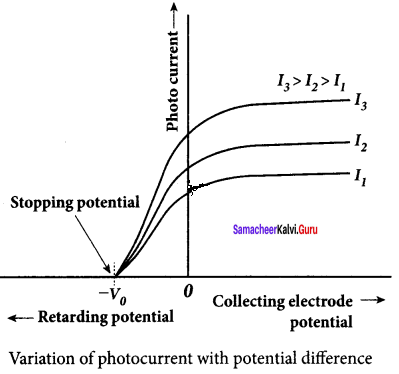6. Stopping potential is that the value of the negative (retarding) potential given to the collecting electrode A which is just sufficient to stop the most energetic photoelectrons emitted and make the photocurrent zero.

7. At the stopping potential, even the most energetic electron is brought to rest. Therefore, the initial kinetic energy of the fastest electron (Kmax ) is equal to the work done by the stopping potential to stop it (eV0 ).
Kmax = $$\frac { 1 }{ 2 }$$ $${ mv }_{ max }^{ 2 }$$ = eV0 …. (1)
Where vmax is the maximun speed of the emitted photoelectron.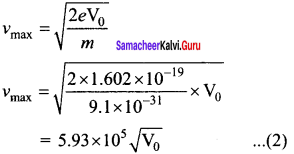= 5.93 x 105 $$\sqrt { { V }_{ 0 } }$$ …. (2)
From equation (1),
Kmax = eVo (in joule) (or) Kmax = (V0 ) (in eV)

8. From the graph, when the intensity of the incident light alone is increased, the saturation current also increases but the value of V0 remains constant.

9. Thus, for a given frequency of the incident light, the stopping potential is independent of intensity of the incident light. This also implies that the maximum kinetic energy of the photoelectrons is independent of intensity of the incident light.

Question 4.
Explain how frequency of incident light varies with stopping potential.
Effect of frequency of incident light on stopping potential:
1. To study the effect of frequency of incident light on stopping potential, the intensity of the incident light is kept constant. The variation of photocurrent with the collector electrode potential is studied for radiations of different frequencies and a graph drawn between them. From the graph, it is clear that stopping potential vary over different frequencies of incident light.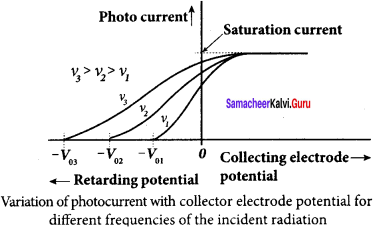2. Greater the frequency of the incident radiation, larger is the corresponding stopping potential. This implies that as the frequency is increased, the photoelectrons are emitted with greater kinetic energies so that the retarding potential needed to stop the photoelectrons is also greater.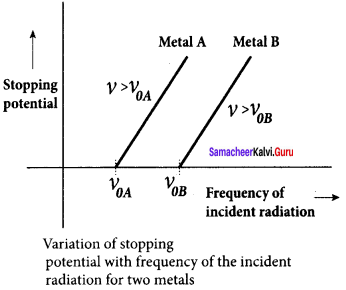3. Now a graph is drawn between frequency and the stopping potential for different metals. From this graph, it is found that stopping potential varies linearly with frequency. Below a certain frequency called threshold frequency, no electrons are emitted; hence stopping potential is zero for that reason. But as the frequency is increased above threshold value, the stopping potential varies linearly with the frequency of incident light.

Question 5.
List out the laws of photoelectric effect.
Laws of photoelectric effect:
1. For a given frequency of incident light, the number of photoelectrons emitted is directly proportional to the intensity of the incident light. The saturation current is also directly proportional to the intensity of incident light.

2. Maximum kinetic energy of the photo electrons is independent of intensity of the incident light.

3. Maximum kinetic energy of the photo electrons from a given metal is directly proportional to the frequency of incident light.

4. For a given surface, the emission of photoelectrons takes place only if the frequency of incident light is greater than a certain minimum frequency called the threshold frequency.

5. There is no time lag between incidence of light and ejection of photoelectrons.

Question 6.
Explain why photoelectric effect cannot be explained on the basis of wave nature of light.
Failures of classical wave theory:
From Maxwell’s theory, light is an electromagnetic wave consisting of coupled electric and magnetic oscillations that move with the speed of light and exhibit typical wave behaviour. Let us try to explain the experimental observations of photoelectric effect using wave picture of light.

1. When light is incident on the target, there is a continuous supply of energy to the electrons. According to wave theory, light of greater intensity should impart greater kinetic energy to the liberated electrons (Here, Intensity of light is the energy delivered per unit area per unit time). But this does not happen. The experiments show that maximum kinetic energy of the photoelectrons does not depend on the intensity of the incident light.

2. According to wave theory, if a sufficiently intense beam of light is incident on the surface, electrons will be liberated from the surface of the target, however low the frequency of the radiation is. From the experiments, we know that photoelectric emission is not possible below a certain minimum frequency. Therefore, the wave theory fails to explain the existence of threshold frequency.

3. Since the energy of light is spread across the wavefront, the electrons which receive energy from it are large in number. Each electron needs considerable amount of time (a few hours) to get energy sufficient to overcome the work function and to get liberated from the surface. But experiments show that photoelectric emission is almost instantaneous process (the time lag is less than 10“9 s after the surface is illuminated) which could not be explained by wave theory.

Question 7.
Explain the quantum concept of light.
Concept of quantization of energy:
Max Planck proposed quantum concept in 1900 in order to explain the thermal radiations emitted by a black body and the shape of its radiation curves. According to Planck, matter is composed of a large number of oscillating particles (atoms) which vibrate with different frequencies. Each atomic oscillator – which vibrates with its characteristic frequency – emits or absorbs electromagnetic radiation of the same frequency. It also says that

1. If an oscillator vibrates with frequency v, its energy can have only certain discrete values, given by the equation.
En = nhυ n = 1, 2, 3 ………..
where A is a constant, called Planck’s constant.

2. The oscillators emit or absorb energy in small packets or quanta and the energy of each quantum is E = hυ.
This implies that the energy of the oscillator is quantized – that is, energy is not continuous as believed in the wave picture. This is called quantization of energy.

Question 8.
Obtain Einstein’s photoelectric equation with necessary explanation. Einstein’s explanation of photoelectric equation:
1.  When a photon of energy hv is incident on a metal surface, it is completely absorbed by a single electron and the electron is ejected.

2.  In this process, a part of the photon energy is used for the ejection of the electrons from the metal surface (photoelectric work function Φ0) and the remaining energy as the kinetic energy of the ejected electron. From the law of conservation of energy,
hυ = Φ0 + $$\frac { 1 }{ 2 }$$ mv2 …… (1)
where m is the mass of the electron and u its velocity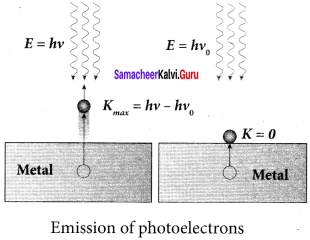3. If we reduce the frequency of the incident light, the speed or kinetic energy of photo electrons is also reduced. At some frequency V0 of incident radiation, the photo electrons are ejected with almost zero kinetic energy. Then the equation (1) becomes
0 = Φ0
where vQ is the threshold frequency. By rewriting the equation (1), we get
hυ = hυ0 + $$\frac { 1 }{ 2 }$$ mv2 …… (2)
The equation (2) is known as Einstein’s Photoelectric equation.
If the electron does not lose energy by internal collisions, then it is emitted with maximum kinetic energy Kmax. Then
Kmax = $$\frac { 1 }{ 2 }$$ $${ mv }_{ max }^{ 2 }$$
where nmax is the maximum velocity of the electron ejected. The equation (1) is rearranged as follows:
Kmax = hυ – Φ0

Question 9.
Explain experimentally observed facts of photoelectric effect with the help of Einstein’s explanation.
Explanation for the photoelectric effect:
The experimentally observed facts of photoelectric effect can be explained with the help of . Einstein’s photoelectric equation.

1. As each incident photon liberates one electron, then the increase of intensity of the light (the number of photons per unit area per unit time) increases the number of electrons emitted thereby increasing the photocurrent. The same has been experimentally observed.

2. From Kmax = hυ – Φ0, it is evident that Kmax is proportional to the frequency of the light and is independent of intensity of the light.

3.  As given in Einstein’s photoelectric equation, there must be minimum energy (equal to the work function of the metal) for incident photons to liberate electrons from the metal surface. Below which, emission of electrons is not possible. Correspondingly, there exists minimum frequency called threshold frequency below which there is no photoelectric emission.

4. According to quantum concept, the transfer of photon energy to the electrons is instantaneous so that there is no time lag between incidence of photons and ejection of electrons.

Question 10.
Give the construction and working of photo emissive cell.
Photo emissive cell:
Its working depends on the electron emission from a metal cathode due to irradiation of light or other radiations.
Construction:
1. It consists of an evacuated glass or quartz bulb in which two metallic electrodes – that is, a cathode and an anode are fixed.

2. The cathode C is semi-cylindrical in shape and is coated with a photo sensitive material. The anode A is a thin rod or wire kept along the axis of the semi-cylindrical cathode.

3. A potential difference is applied between the anode and the cathode through a galvanometer G.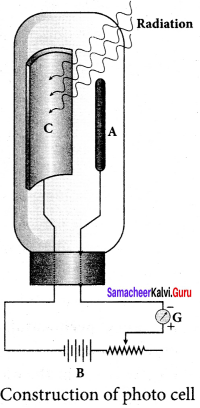Working:
4.  When cathode is illuminated, electrons are emitted from it. These electrons are attracted by anode and hence a current is produced which is measured by the galvanometer.

5. For a given cathode, the magnitude of the current depends on
(i) the intensity to incident radiation and (ii) the potential difference between anode and cathode.

Question 11.
Derive an expression for de Broglie wavelength of electrons.
An electron of mass m is accelerated through a potential difference of V volt. The kinetic
energy acquired by the electron is given by
$$\frac { 1 }{ 2 }$$ mv2 = evacuated
Therefore, the speed v of the electron is v = $$\sqrt { \frac { 2eV }{ m } }$$
Hence, the de Broglie wavelength of the electron is λ = $$\frac { h }{ mv }$$ = $$\frac { h }{ \sqrt { 2emV } }$$
Substituting the known values in the above equation, we get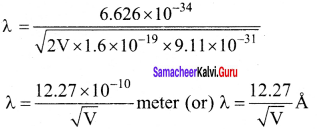For example, if an electron is accelerated through a potential difference of 100V, then its de Broglie wavelength is 1.227 A. Since the kinetic energy of the electron, K = eV, then the de Broglie wavelength associated with electron can be also written as
λ = $$\frac { h }{ \sqrt { 2mK } }$$

Question 12.
Briefly explain the principle and working of electron microscope.
Electron Microscope:
Principle:
1. This is the direct application of wave nature of particles. The wave nature of the electron is used in the construction of microscope called electron microscope.

2. The resolving power of a microscope is inversely proportional to the wavelength of the radiation used for illuminating the object under study. Higher magnification as well as higher resolving power can be obtained by employing the waves of shorter wavelengths.

3. De Broglie wavelength of electron is very much less than (a few thousands less) that of the visible light being used in optical microscopes.

4. As a result, the microscopes employing de Broglie waves of electrons have very much higher resolving power than optical microscope.

5. Electron microscopes giving magnification more than 2,00.000 times are common in research laboratories.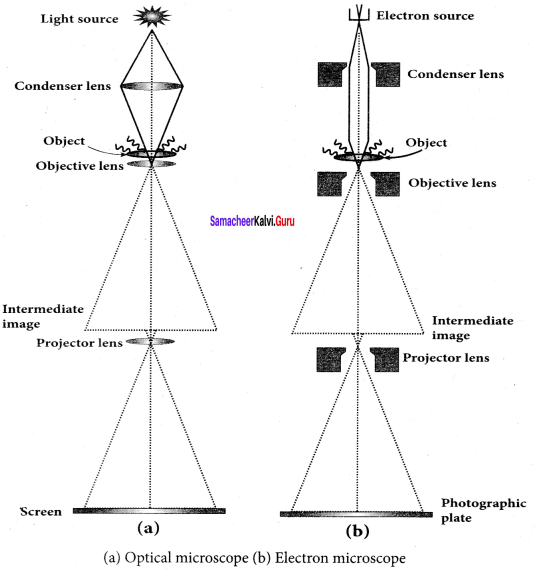Working:
1. The electron beam passing across a suitably arranged either electric or magnetic fields undergoes divergence or convergence thereby focussing of the beam is done.

2. The electrons emitted from the source are accelerated by high potentials. The beam is made parallel by magnetic condenser lens. When the beam passes through the sample whose magnified image is needed, the beam carries the image of the sample.

3. With the help of magnetic objective lens and magnetic projector lens system, the magnified image is obtained on the screen. These electron microscopes are being used in almost all branches of science.

Question 13.
Describe briefly Davisson – Germer experiment which demonstrated the wave nature of electrons.
Davisson – Germer experiment:
1. De Broglie hypothesis of matter waves was experimentally confirmed by Clinton Davisson and Lester Germer in 1927. They demonstrated that electron beams are diffracted when they fall on crystalline solids.

2. Since ciystal can act as a three-dimensional diffraction grating for matter waves, the electron waves incident on crystals are diffracted off in certain specific directions.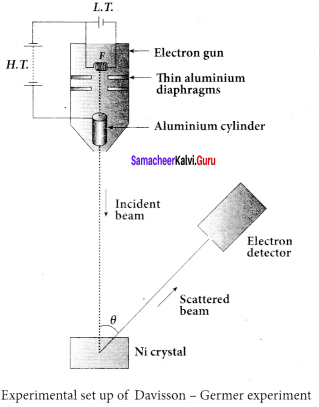3. The filament F is heated by a low tension (L.T.) battery. Electrons are emitted from the hot filament by thermionic emission. They are then accelerated due to the potential difference between the filament and the anode aluminium cylinder by a high tension (H.T.) battery.

4. Electron beam is collimated by using two thin aluminium diaphragms and is allowed to strike a single crystal of Nickel.

5. The electrons scattered by Ni atoms in different directions are received by the electron detector which measures the intensity of scattered electron beam.

6. The detector is rotatable in the plane of the paper so that the angle Φ between the incident
beam and the scattered beam can be changed at our will.

7. The intensity of the scattered electron beam is measured as a function of the angle θ.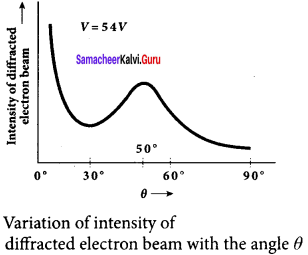8. From the graph shows the variation of intensity of the scattered electrons with the angle 0 for the accelerating voltage of 54V. For a given accelerating voltage V, the scattered wave shows a peak or maximum at an angle of 50° to the incident electron beam.

This peak in intensity is attributed to the constructive interference of electrons diffracted from various atomic layers of the target material.

9. From the known value of interplanar spacing of Nickel, the wavelength of the electron wave has been experimentally calculated as 1.65 Å.

10. The wavelength can also be calculated from de Broglie relation for V = 54 V from equation.
λ = $$\frac { 12.27 }{ \sqrt { V } }$$ Å = $$\frac { 12.27 }{ \sqrt { 54 } }$$
λ = 1.67 Å

11. This value agrees well with the experimentally observed wavelength of 1.65 Å. Thus this experiment directly verifies de Broglie’s hypothesis of the wave nature of moving particles.

Samacheer Kalvi 12th Physics Dual Nature of Radiation and Matter Numerical problems

Question 1.
How many photons per second emanate from a 50 mW laser of 640 nm?
P = 50 mW
λ = 640 nm
h = 6.6 x 10-34 Js
c = 3 x 108 ms-1
Number of photons emanate per second np = $$\frac { P }{ E }$$ =$$\frac { Pλ }{ hc }$$
= $$\frac { 50\times { 10 }^{ 3 }\times 640\times { 10 }^{ -9 } }{ 6.6\times { 10 }^{ -34 }3\times { 10 }^{ 8 } }$$ = $$\frac { 32000\times { 10 }^{ -6 } }{ 19.8\times { 10 }^{ -26 } }$$ = 1616.16 x 10-6
np = 1.61 x 101017 s-1

Question 2.
Calculate the maximum kinetic energy and maximum velocity of the photoelectrons emitted when the stopping potential is 81 V for the photoelectric emission experiment.
V0 = 81 V
e= 1.6 x 10-19 C
m = 9.1 x 10-31 kg
Maximum kinetic energy of electron,
Kmax = eVo
= 1.6 x 10-19 x 81
= 129.6 x 10-19
= 1.29 x 10-17
Kmax = 1.3 x 10-17 J
aximum velocity of photoelectron,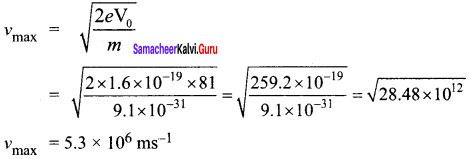Question 3.
Calculate the energies of the photons associated with the following radiation:
(i) violet light of 413 nm
(ii) X-rays of 0.1 nm
(iii) radio waves of 10 m.
h = 6.6 x 10-34 Js
c = 3 x 108 ms-1
Energy of photon, E = hυ
E = $$\frac { hc }{ λ }$$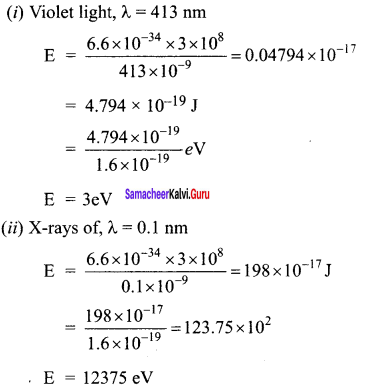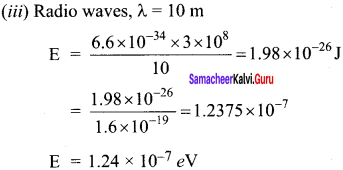Question 4.
A 150 W lamp emits light of mean wavelength of 5500 Å . If the efficiency is 12%, find out the number of photons emitted by the lamp in one second.
P= 150W
λ = 5500 Å
h = 6.6 x 10-34 Js
c = 3 x 108 ms-1
Number of photons emitted per second n = $$\frac { pλ }{ hc }$$
If the efficiency is 12%, η = $$\frac { 12 }{ 100 }$$ = 0.12
n = $$\frac { pηλ }{ hc }$$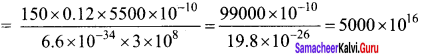n = 5 x 1019

Question 5.
How many photons of frequency 1014 Hz will make up 19.86 J of energy?
Total energy emitted per second = Power x time
19.86 J = Power x is
∴ Power = 19.86 W
Number of photons, n = $$\frac { p }{ E }$$ = $$\frac { p }{ hυ }$$
= $$\frac { 19.86 }{ 6.6\times { 10 }^{ -34 }\times { 10 }^{ 14 } }$$ = 3.009 x 1020
n = 3 x 1020
np = 3 x 1020

Question 6.
What should be the velocity of the electron so that its momentum equals that of 4000 Å wavelength photon.
de-Broglie wavelength of electron
λ = $$\frac { h }{ p }$$
v = $$\frac { h }{ mλ }$$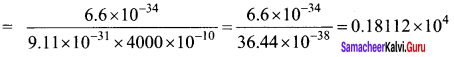v = 1811 ms-1

Question 7.
When a light of frequency 9 x 1014 Hz is incident on a metal surface, photoelectrons are emitted with a maximum speed of 8 x 105ms-1. Determine the threshold frequency of the surface.
According to Einstein’s photoelectric equation
$$\frac { 1 }{ 2 }$$ $${ mv }_{ max }^{ 2 }$$ = h (υ-υ0)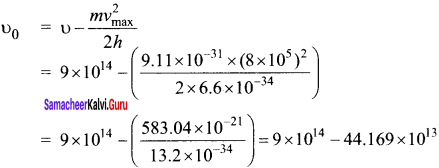= 9 x 014-4.4 x 1014
υ0 = 4.6 x 1014 Hz

Question 8.
When a 6000 Å light falls on the cathode of a photo cell and produced photoemission. If a stopping potential of 0.8 V is required to stop emission of electron, then determine the:

1. frequency of the light
2. energy of the incident photon
3. work function of the cathode material
4. threshold frequency
5. net energy of the electron after it leaves the surface.

Wavelength, λ = 6000 Å= 6000 x 10-10 m
stopping potential, V0 = 0.8 V
1. Frequency of the light, υ = $$\frac { c }{ λ }$$
= $$\frac { 3\times { 10 }^{ 8 } }{ 600\times { 10 }^{ -10 } }$$ = 5 x 104 x 10-18
υ = 5 x 1014 Hz

2. Energy of the incident photon,
E = hυ = 6.6 x 10-34 x 5 x 1014
= 33 x 10-20 J
= $$\frac { 33\times { 10 }^{ -20 } }{ 1.6\times { 10 }^{ -19 } }$$ = 20.625 x 10-1
E = 2.06 eV

3. Work function of the cathode material.
W0 = hυ – eV0
= $$\left(\frac{6.6 \times 10^{-34} \times 5 \times 10^{14}}{1.6 \times 10^{-19}}\right)$$ – $$\left(\frac{1.6 \times 10^{-19} \times 0.8}{1.6 \times 10^{-19}}\right)$$ = 2.06-0.8
W0 = 1.26 eV

4. Threshold frequency, W0 = hυ0
υ0 = $$\frac{W_{0}}{h}$$ = $$\frac{1.26 \times 1.6 \times 10^{-19}}{6.6 \times 10^{-34}}$$ = 0.3055 x 1015
υ0 = 3.05 x 1014 Hz

5. Net energy of the electron after it leaves the surface
E = (υ – υ0)
= 6.6 x 10-34 (5 x1014 – 3.06 x 1014
= 6.6 x 10-34 x 1.94 x 1014
E = 12.804 x 10-20 J
= $$\frac{1.2804 \times 10^{-19}}{1.6 \times 10^{-19}}$$
E = 0.8 e V

Question 9.
A 3310 Å photon liberates an electron from a material with energy 3 x 10-19 J while another 5000 Å photon ejects an electron with energy 0.972×10-19 J from the same material. Determine the value of Planck’s constant and the threshold wavelength of the material.
They energy of ejected electron is given by E = $$\frac { hc }{ λ }$$ – $$\frac { hc }{ { \lambda }_{ 0 } }$$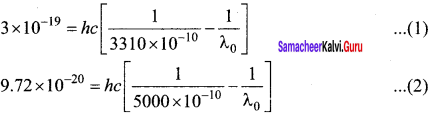Subtracting (2) from (1), we get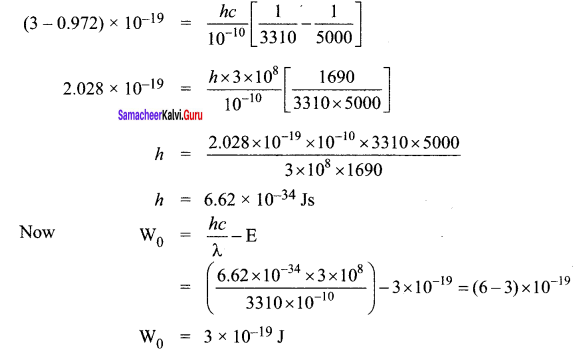Threshold Wavelength,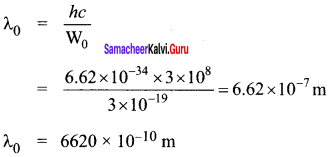Question 10.
At the given point of time, the earth receives energy from Sun at 4 cal cm-2 min-1. Determine the number of photons received on the surface of the Earth per cm2 per minute. (Given : Mean wavelength of Sun light = 5500 Å)
E= 4 calorie
= 4 x 4.184 J
λ = 5500 Å
Number of photons received on the surface of the earth, from E = nhυ
n = $$\frac { E λ}{ hc }$$
= $$\frac{4 \times 4.184 \times 5500 \times 10^{-10}}{6.6 \times 10^{-34} \times 3 \times 10^{8}}$$ = $$\frac{9.2048 \times 10^{-10}}{19.8 \times 10^{-26}}$$ = 4648 x 1016
= 4.648 x 1019
n = 4.65 x 1019

Question 11.
UV light of wavelength 1800 Å is incident on a lithium surface whose threshold wavelength 4965 Å. Determine the maximum energy of the electron emitted.
λ = 1800 x 10-10 m
λ0 = 4965 x 10-10m
h = 6.6 x 10-34 Js
c = 3 x 108 ms-1
Maximum kinetic energy of electron,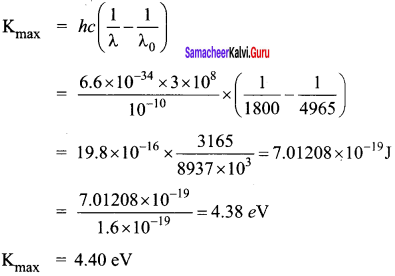Question 12.
Calculate the de Broglie wavelength of a proton whose kinetic energy is equal to 81.9 x 10-15 J. (Given: mass of proton is 1836 times that of electron).
mp = 1.67 x 10-27 kg
K.E = 81.9 x 10-15 J
de-Broglie wavelength of proton, λ = $$\frac { h }{ \sqrt { 2mK } }$$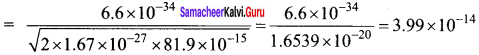λ = 4 x 10-14 m

Question 13.
A deuteron and an alpha particle are accelerated with the same potential. Which one of the two has (i) greater value of de Broglie wavelength associated with it and (ii) less kinetic energy? Explain.
(i) Using de-Broglie wavelength formula, the dueteron and alpha particle are accelerated with same potential. So, both their velocities are same.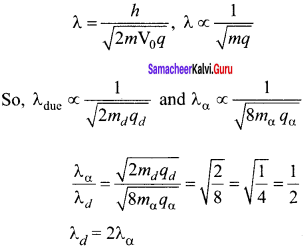(ii) For same potential of acceleration, KE is directly proportional to the ‘q’
Charge of duetron is +e
Charge of alpha is +2e
So, Kd = $$\frac {{ K }_{α}}{ 2 }$$
Charge of alpha particle is more than the duetron.

Question 14.
An electron is accelerated through a potential difference of 81V. What is the de Broglie wavelength associated with it? To which part of electromagnetic spectrum does this wavelength correspond?
de-Broglie wavelength of an electron beam accelerated through a potential difference of V volts is
λ = $$\frac { h }{ \sqrt { 2meV } }$$ = $$\frac { 1.23 }{ \sqrt { V } }$$ nm
V = 81 V, so λ = $$\frac { 1.23 }{ \sqrt { 81 } }$$ x 10-9 m
λ = 1.36 Å
X-ray is the part of electromagnetic spectrum does this wavelength corresponds. X-ray has the wavelengths ranging from about 108 to 10-12 m.

Question 15.
The ratio between the de Broglie wavelengths associated with protons, accelerated through a potential of 512 V and that of alpha particles accelerated through a potential of X volts is found to be one. Find the value of X.
de-Broglie wavelength of accelerated charge particle
λ = $$\frac { h }{ \sqrt { 2mqV } }$$
λ ∝ $$\frac { h }{ \sqrt { mqV } }$$
Ratio of wavelength of proton and a-particle.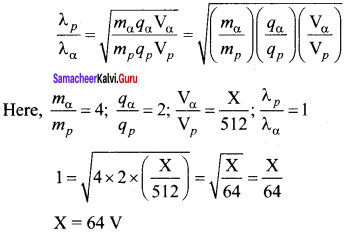### Samacheer Kalvi 12th Physics Dual Nature of Radiation and Matter Additional Questions

Samacheer Kalvi 12th Physics Dual Nature of Radiation and Matter Multiple Choice Questions

Question 1.
The maximum kinetic energy of photoelectrons emitted from a surface when photons of
energy 3 eV fall on it is 4 eV. The stopping potential, in volt, is
(a) 2
(b) 4
(c) 6
(d) 10
(b) 4
Hint:
Stopping potential, V0 = $$\frac { { K }_{ max } }{ e }$$ = $$\frac { 4eV }{ e }$$ = 4v

Question 2.
If an electron and proton are propagating in the form of waves having the same λ, it implies that they have the same-
(a) energy
(b) momentum
(c) velocity
(d) angular momentum
(b) momentum
Hint: Momentum, p = $$\frac { h }{ λ }$$
As both electron and proton have same λ, so they have the same momentum

Question 3.
An electron of mass m and charge e is accelerated from rest through a potential difference V in vacuum. Its final velocity will be-
(a) $$\sqrt { \frac { 2eV }{ m } }$$
(b) $$\sqrt { \frac { eV }{ m } }$$
(c) $$\frac { ev }{ 2m }$$
(d) $$\frac { ev }{ m }$$
(a) $$\sqrt { \frac { 2eV }{ m } }$$
Hint:
K.E. gained by an electron when accelerated through a potential difference V,
$$\frac { 1 }{ 2 }$$ mv2 = eV or v = $$\sqrt { \frac { 2eV }{ m } }$$

Question 4.
The work function of a substance is 4.0 eV. The longest wavelength of light that can cause photoelectron emission from this substance is approximately
(a) 540 nm
(b) 400 nm
(c) 310 nm
(d) 220 nm
(c) 310 nm
Hint:
λ0 = $$\frac { hc }{ W }$$ = $$\frac{6.63 \times 10^{-34} \times 3 \times 10^{8}}{4.0 \times 1.6 \times 10^{-19}}$$ = m = 310 x 10-9 m = 310 nm

Question 5.
Sodium and copper have work function 2.3 eV and 4.5 eV respectively. Then, the ratio of their threshold wavelength is nearest to-
(a) 1 : 2
(b) 4 : 1
(c) 2 : 1
(d) 1 : 4
(c) 2 : 1
Hint:
$$\frac{\lambda_{0}(\mathrm{Na})}{\lambda_{0}(\mathrm{Cu})}$$ = $$\frac{\mathrm{W}_{0}(\mathrm{Cu})}{\mathrm{W}_{0}(\mathrm{Na})}$$

Question 6.
The surface of a metal is illuminated with the light of 400 nm. The kinetic energy of the ejected photoelectrons was found to be 1.68 eV. The work function of the metal is (hc = 1240 eV nm)
(a) 3.09 eV
(b) 1.41 eV
(c) 1.51 eV
(d) 1.68 eV
Anwer:
(b) 1.41 eV
Hint:
Kmax = $$\frac { hc }{ λ }$$ W0 or W0 = $$\frac { hc }{ λ }$$ – Kmax
= $$\frac { 1240 }{ 400 }$$-1.68 = 3.10-1.68 = 1.42ev

Question 7.
4 eV is the energy of the incident photon and the work function is 2 eV. The stopping potential will be
(a) 2V
(b) 4V
(c) 6V
(d) 2√2V
(d) 2√2V
Hint:
eV0= hv- W0 = 4eV – 2eV = 2eV
∴ V0= $$\frac { 2ev }{ e }$$ = 2v

Question 8.
A light having wavelength 300 nm falls on a metal surface work function of metal is 2.54 eV. What is stopping potential?
(a) 1.4 V
(b) 2.59 V
(c) 1.60 V
(d) 1.29 V
(a) 1.4 V
Hint:
eV0 = hu – W0 = 2eV – 0.6 eV = 1.4 eV
∴ V0= $$\frac { 1.4eV }{ e }$$ = 1.4eV

Question 9.
If the kinetic energy of a free electron doubles, its de-Broglie wavelength changes by the factor
(a) $$\frac { 1 }{ 2 }$$
(b) 2
(c) $$\frac { 1 }{ √2 }$$
(d) √2
(c) $$\frac { 1 }{ √2 }$$
Hint:
λ = $$\frac{h}{\sqrt{2 m \mathrm{K}}}$$
When kinetic energy is doubled, λ’ = $$\frac{h}{\sqrt{2 m \times 2 K}}$$ = $$\frac { 1 }{ √2 }$$λ

Question 10.
If the kinetic energy of a particle is increased by 16 times, the percentage change in the de-Broglie wavelength of the particle is
(a) 25%
(b) 75%
(c) 60%
(d) 50%
(b) 75%
Hint:
λ = $$\frac{h}{\sqrt{2 m \mathrm{K}}}$$ ; $$\frac{h}{\sqrt{2 m \times 16 K}}$$ = $$\frac { λ }{ 4 }$$
% change in de-Broglie wavelength, $$\frac { λ-λ’ }{ λ }$$ = [1-$$\frac { λ }{ λ’ }$$] x 100 [1-$$\frac { 1 }{ 4 }$$] x 100 = 75%

Question 11.
When a proton is accelerated through IV, then its kinetic energy will be
(a) 1 eV
(b) 13.6 eV
(c) 1840 eV
(d) 0.54 eV
(a) 1 eV
Hint:
K = qV = e x 1V= 1 eV

Question 12.
The kinetic energy of an electron, which is accelerated in the potential difference of 100 volts, is
(a) 416.6 cal
(b) 6.636 cal
(c) 1.602 x 10-17 J
(d) 1.6 x 104 J
(c) 1.602 x 10-17 J
Hint:
K = eV = 1.602 x 10(c) 1.602 x 10-19 x 100 J
= 1.602 x 10(c) 1.602 x 10-17 J

Question 13.
Kinetic energy of emitted electron depends upon
(a) frequency
(b) intensity
(c) nature of atmosphere surrounding the electron
(d) none of these
(a) frequency
Hint:
Kinetic energy of emitted electron depends on the frequency of incident radiation.

Question 14.
The work function of photometal is 6.626 eV. What is the threshold wavelength?
(a) 3921 Å
(b) 1875 Å
(c) 1867 Å
(d) 4433 Å
(b) 1875 Å
Hint:
λ0 = $$\frac { hc }{{ W }_{ 0 }}$$ = $$\frac{6.63 \times 10^{-34} \times 3 \times 10^{8} \times 10^{10}}{6.626 \times 1.6 \times 10^{-19}}$$ Å = 1875 Å

Question 15.
The number of photo-electrons emitted for light of a frequency υ (higher than the threshold frequency υ0) is proportional to
(a) Threshold frequency (υ0)
(b) Intensity of light
(c) Frequency of light (υ)
(d) υ – υ0
(b) Intensity of light
Hint:
Photoelectric current oc Intensity of incident light

Question 16.
The speed of an electron having a wavelength of 10-10 m is
(a) 7.25 x 106 ms-1
(b) 6.26 x 106 ms-1
(c) 5.25 x 106 ms-1
(d) 4.24 x 106 ms-1
(a) 7.25 x 106 ms-1
Hint:
As λ = $$\frac { h }{ mv }$$
∴ v = $$\frac { h }{ mλ }$$ = $$\frac{6.6 \times 10^{-34}}{9.1 \times 10^{-31} \times 10^{-10}}$$ = 7.25 x 106 ms-1

Question 17.
If an electron and a photon propagate in the form of waves having the same wavelength, it implies that they have the same
(a) energy
(b) momentum
(c) angular momentum
(d) velocity
(b) momentum
Hint:
As both electron and photon have same de-Broglie wavelength (λ = h /p), so they have the same momentum P.

Question 18.
Electron volt is a unit of
(a) Energy
(b) potential
(c) current
(d) charge
(a) Energy
Hint:
Electron volt is a unit of energy

Question 19.
Photon of frequency u has a momentum associated with it. If c is the velocity of radiation, then the momentum is
(a) $$\frac { hυ }{ c }$$
(b) $$\frac { υ }{ c }$$
(c) hυc
(d) $$\frac { h }{ { c }^{ 2 } }$$
(a) $$\frac { hυ }{ c }$$
Hint:
P = $$\frac { E }{ { c }^{ 2 } }$$ = $$\frac { hυ }{ c }$$

Question 20.
The time taken by a photoelectron to come out after photon strikes is approximately
(a) 10-14 s
(b) 10-10 s
(c) 10-16 s
(d) 10-1 s
(b) 10-10 s
Hint:
The time lag between the incident of photon and the emission of photoelectrons is 10-10 s approximately.

Question 21.
Cathode rays consist of
(a) photons
(b) electrons
(c) protons
(d) α-particles
(b) electrons

Question 22.
The momentum of photon whose frequency is f is
(a) $$\frac { hf }{ c }$$
(b) $$\frac { hc }{ f }$$
(c) $$\frac { h }{ f }$$
(d) $$\frac { c }{ hf }$$
(a) $$\frac { hf }{ c }$$
Hint:
p = mc = $$\frac { { mc }^{ 2 } }{ { c } }$$ = $$\frac { hf }{ c }$$

Question 23.
The energy of photon of wavelength λ is
(a) $$\frac { hc }{ λ }$$
(b) hλc
(c) $$\frac { λ }{ hc }$$
(d) $$\frac { hλ }{ c }$$
(a) $$\frac { hc }{ λ }$$
Hint:
E = hυ = $$\frac { hc }{ λ }$$

Question 24.
The ratio of the energy of a photon with λ = 150 nm to that with λ = 300 nm is
(a) 2
(b) $$\frac { 1 }{ 4 }$$
(c) 2
(d) $$\frac { 1 }{ 2 }$$
(a) 2
Hint:
$$\frac {{ E }_{ 1 }}{ { E }_{ 2 } }$$ = $$\frac {{ λ }_{ 2 }}{ { λ }_{ 1 } }$$ = $$\frac { 300 }{ 150 }$$ = 2

Question 25.
Photons of 5.5 eV energy fall on the surface of the metal emitting photoelectrons of maximum kinetic energy 4.0 eV. The stopping voltage required for these electrons is
(a) 5.5 V
(b) 1.5 V
(c) 9.5 V
(d) 4.0 V
(d) 4.0 V
Hint:
Stopping potential = $$\frac { { K }_{ max } }{ e }$$ = $$\frac { 4.0ev }{ e }$$ = 4.0V

Question 26.
The wavelength of photon is proportional to (where υ = frequency)
(a) υ
(b) √υ
(c) $$\frac { 1 }{ √υ }$$
(d) $$\frac { 1 }{ υ }$$
(d) $$\frac { 1 }{ υ }$$
Hint:
λ = $$\frac { c }{ υ }$$ i.e., λ ∝ $$\frac { 1 }{ υ }$$

Question 27.
What is the energy of a photon whose wavelength is 6840 Å?
(a) 1.81 eV
(b) 3.6 eV
(c) – 13.6 eV
(d) 12.1 eV
(a) 1.81 eV
Hint:
E = hυ = $$\frac { hc }{ λ }$$ = $$\frac { 12400ev Å }{ 8840 Å }$$ = 1.81 eV

Question 28.
Momentum of photon of wavelength λ is
(a) $$\frac { hυ }{ c }$$
(b) zero
(c) $$\frac { hλ }{{ c }^{ 2 }}$$
(d) $$\frac { hλ }{c}$$
(a) $$\frac { hυ }{ c }$$
Hint:
p = mc = $$\frac {{ mc }^{ 2 }}{c}$$ = $$\frac { hυ }{ c }$$

Question 29.
The momentum of a photon of energy 1 MeV in kg m/s will be
(a) 5 x 10-22
(b) 0.33 x 106
(c) 7 x 10-24
(d) 10-22
(a) 5 x 10-22
Hint:
P = $$\frac { E }{ c }$$ = $$\frac{1 \mathrm{MeV}}{3 \times 10^{8} \mathrm{ms}^{-1}}$$ = $$\frac{1.6 \times 10^{-13} \mathrm{J}}{3 \times 10^{8} \mathrm{ms}^{-1}}$$ = 5.33 x 10-22 Kg ms-1

Question 30.
If we consider electrons and photons of same wavelength then will have same
(a) momentum
(b) angular momentum
(c) energy
(d) velocity
(a) momentum
Hint:
As p = h/λ, so electrons and photons having the same wavelength λ will have the same momentum p.

Question 31.
Photoelectric effect can be explained by
(a) corpusular theory of light
(b) wave nature of light
(c) Bohr’s theory
(d) quantum theory of light
(d) quantum theory of light

Question 32.
Which of the following waves can produce photoelectric effect?
(a) ultrasound
(b) infrared
(d) X-rays
(d) X-rays
Hint:
Electromagnetic radiation, being of high frequency such as X-rays can produce photoelectric effect.

Question 33.
Which light when falls on a metal will emit photoelectrons?
(d) microwaves
Hint:
Ultraviolet radiation, being of high frequency, can emit photoelectrons from metals.

Question 34.
In photoelectric effect, the KE of electrons emitted from the metal surface depends upon
(a) intensity of light
(b) frequency of incident light
(c) velocity of incident light
(d) both intensity and velocity of light
(b) frequency of incident light
Hint:
The kinetic energy of photoelectrons depends upon the frequency of incident light.

Question 35.
In photoelectric effect, electrons are ejected from metals, if the incident light has a certain minimum
(a) wavelength
(b) frequency
(c) amplitude
(d) angle of incidence
(b) frequency
Hint:
For photoelectric emission, the incident light must have a certain minimum frequency, called threshold frequency.

Question 36.
Number of ejected photoelectrons increases with increases
(a) in intensity of light
(b) in wavelength of light
(c) in frequency of light
(d) never
(a) in intensity of light
Hint:
Number of ejected photoelectrons increases with the increase in intensity of light.

Question 37.
By photoelectric effect, Einstein proved
(a) E = hυ
(b) K.E. = $$\frac { 1 }{ 2 }$$mv2
(c) E = mc2
(d) E = $$\frac {{ -Rhc }^{ 2 }}{{ n }^{ 2 }}$$
(a) E = hυ
Hint:
Einstein explained photoelectric effect on the basis of planck’s quantum theory of radiation and hence supported the relation : E = hυ

Question 38.
A photocell employs photoelectric effect to convert
(a) change in the frequency of light into a change in the electric current
(b) Change in the frequency of light into a change in electric voltage
(c) Change in the intensity of illumination into a change in photoelectric current
(d) Change in the intensity of illumination into a change in the work function of the photo cathode
(c) Change in the intensity of illumination into a change in photoelectric current
Hint:
It indicates that threshold frequency is greater than that of ultraviolet light. As X-rays have greater frequency than uv rays, so they can cause photoelectric effect.

Question 39.
When ultraviolet rays incident on metal plate there photoelectric effect does not occur, it occurs by incident of
(a) infrared rays
(b) X-rays
(d) microwave
(b) X-rays
Hint:
It indicates that threshold frequency is greater than that of ultraviolet light. As X-rays have greater frequency than UV rays, so they can cause photoelectric effect.

Question 40.
The threshold frequency for photoelectric effect on sodiune corresponds to a wavelength of 5000 Å. Its function is
(a) 4 x 10-19 J
(b) 1J
(c) 2 x 10-19 J
(d) 3 x 10-19 J
(a) 4 x 10-19 J
Hint:
W0 = $$\frac { hc }{{ λ }_{ 0 }}$$ = $$\frac{6.63 \times 10^{-34} \times 3 \times 10^{8}}{5000 \times 10^{-10}}$$ J = 4 x 10-19 J

Question 41.
The photoelectric work function for a metal surface is 4.125 eV. The cut off wavelength for this surface is
(a) 3000 Å
(b) 2062.5 Å
(c) 4125 Å
(d) 6000 Å
(a) 3000 Å
Hint:
λ0 = $$\frac { hc }{{ W }_{ 0 }}$$ = $$\frac{6.63 \times 10^{-34} \times 3 \times 10^{8}}{4.125 \times 1.6 \times 10^{-19}}$$ m = 3 x 10-7 m = 3000 Å

Question 42.
Ultraviolet radiations of 6.2 eV falls on an aluminium surface. Kinetic energy of fastest electrons emitted is (work function = 4.2 eV)
(a) 3.2 x 10-21 J
(b) 3.2 x 10-19 J
(c) 7 x 10-25 J
(d) 9 x 10-32 J
(b) 3.2 x 10-19 J
Hint:
Kmax = hυ- W0 = 6.2 eV – 4.2 eV
= 2.0 eV = 2.0 x 1.6 x 10-19 J =3.2 x 10-19 J

Question 43.
The de-Broglie wavelength of a tennis ball of mass 60g moving with a velocity of 10 ms-1 is approximately (planck’s constant, h = 6.63 x 10-34 Js)
(a) 10-33 m
(b) 10-31 m
(c) 10-16 m
(d) 10-25 m
(a) 10-33 m
Hint:
λ = $$\frac { h }{ mv }$$ = $$\frac{6.63 \times 10^{-34}}{60 \times 10^{-3} \times 10}$$ ≈ 10-33 m

Question 44.
The wavelength of de-Broglie wave is 2 μm, then its momentum (h = 6.63 x 10-34 Js) is
(a) 3.315 x 10-28 kg ms-1
(b) 1.66 x 10-28 kg ms-1
(c) 4.97 x 10-28 kg ms-1
(d) 9.9 x 10-28 kg ms-1
(a) 3.315 x 10-28 kg ms-1
Hint:
p = $$\frac { h }{ λ }$$ = $$\frac{6.03 \times 10^{-34} \mathrm{Js}}{2 \times 10^{-6} \mathrm{m}}$$ = 3.315 x 10-28 kg ms-1

Question 45.
What is de-Broglie wavelength of electron having energy 10 KeV?
(a) 0.12 Å
(b) 1.2 Å
(c) 12.2 Å
(d) none of these
(a) 0.12 Å
Hint:
λ = $$\frac { 12.3 }{ √v }$$ Å = $$\frac { 12.3 }{ \sqrt { 10\times { 10 }^{ 3 } } }$$ = 0.12Å

Question 46.
Which one of the following property does not support wave theory of light?
(a) Light obeys laws of reflection and refraction
(b) Light waves get polarised
(c) Light shows photoelectric effect
(d) Light shows interference
(c) Light shows photoelectric effect
Hint:
Photoelectric effect cannot be explained on the basis of wave theory of light.

Question 47.
de-Broglie wavelength λ associated with neutrons is related with absolute temperature T as
(a) λ ∝ T
(b) λ ∝ $$\frac { 1 }{ T }$$
(c) λ ∝ $$\frac { 1 }{ √T }$$
(d) λ ∝ T2
(c) λ ∝ $$\frac { 1 }{ √T }$$
Hint:
λ = $$\frac { h }{ \sqrt { 2mK } }$$ = $$\frac { h }{ \sqrt { 3mKT } }$$ ⇒ λ ∝ $$\frac { 1 }{ √T }$$

Question 48.
As the intensity of incident light increases
(a) kinetic energy of emitted photoelectrons increases
(b) photoelectric current decreases
(c) photoelectric current increases
(d) kinetic energy of emitted photoelectrons decreases
(c) photoelectric current increases
Hint:
As the intensity of incident light increases, photoelectric current increases.

Question 49.
The de Broglie wave corresponding to a particle of mass m and velocity u has a wavelength associated with it
(a) $$\frac { h }{ mυ }$$
(b) hmυ
(c) $$\frac { mh }{ υ }$$
(d) $$\frac { m }{ hυ }$$
(a) $$\frac { h }{ mυ }$$
Hint:
de-Broglie wavelength, λ = $$\frac { h }{ p }$$ = $$\frac { h }{ mυ }$$

Question 50.
If particles are moving with same velocity, then which has maximum de-broglie wavelength?
(a) Proton
(b) α-particle
(c) Nevtron
(d) β-particle
(d) β-particle
Hint:
As λ = h/mv, of the given particles β – particle is the lightest, so it will have maximum de-Broglie wavelength.

Question 51.
The dual nature of light is exhibited by
(a) diffraction and photoelectric effect
(b) photoelectric effect
(c) refraction and interference
(d) diffraction and reflection
(a) diffraction and photoelectric effect
Hint:
Diffraction exhibits wave nature while photoelectric effect exhibits particle nature. Hence these two phenomena exhibit dual nature of light.

Question 52.
If the momentum of a particle is doubled, then its de-Broglie wavelength will-
(a) remain unchanged
(b) become four time
(c) become two times
(d) become half
(d) become half
Hint:
As λ = $$\frac { h }{ p }$$ when momentum p is doubled, wavelength will become half the initial value.

Question 53.
Moving with the same velocity, which of the following has the longest de-Broglie wavelength?
(a) β – particle
(b) α – particle
(c) proton
(d) neutron
(a) β – particle
Hint:
λ = $$\frac { h }{ mv }$$ λ ∝ $$\frac { 1 }{ m}$$
As β – particle (an electron) has the smallest mass, so it has the longest de-Broglie wavelength.

Question 54.
What is the de-Broglie wavelength of the a-particle accelerated through a potential difference of V volt? (mass of a-particle = 6.6455 x 10-27 kg)
(a) $$\frac { 0.287 }{ √V }$$ Å
(b) $$\frac { 12.27 }{ √V }$$ Å
(c) $$\frac { 0.101 }{ √V }$$ Å
(d) $$\frac { 0.202 }{ √V }$$ Å
(c) $$\frac { 0.101 }{ √V }$$ Å
Hint:
K = qV = 2eV
λ = $$\frac { h }{ \sqrt { 2mK } }$$ = $$\frac { h }{ \sqrt { 2m\times 2eV\quad } }$$ = $$\frac { h }{ \sqrt { 4meV } }$$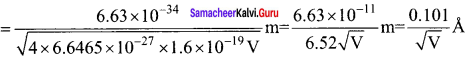Question 55.
A proton and an a – particle are accelerated through the same potential difference. The ratio of de-Broglie wavelength of proton to the de-Broglie wavelength of alpha particle will be
(a) 1 : 2
(b) 2√2 :1
(c) 2 : 1
(d) 1:1
(b) 2√2 :1
Hint: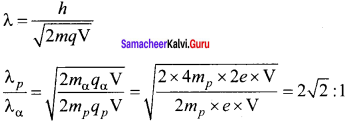Question 56.
Proton and α – particle have the same de-Broglie wavelength. What is same for both of them?
(a) Time period
(b) Energy
(c) Frequency
(d) Momentum
(d) Momentum
Hint:
λ = h/p, when wavelength λ is same, momentump is also same.

Question 57.
The shortest wavelength of X-ray emitted from an X-ray tube depends upon.
(a) the current in the tube
(b) the voltage applied to the tube
(c) the nature of the gas in the tube
(d) the atomic number of the target material
(b) the voltage applied to the tube
Hint:
λmin = $$\frac { hc }{ eV }$$ i.e.,λmin ∝ $$\frac { 1 }{ V }$$

Question 58.
An X-ray tube operates on 30 kV. The minimum wavelength emitted is h = 6.6 x 10-34 Js, c = 3 x 108 m/s, e = 1.6 x 10-19C.
(a) 6.6 Å
(b) 0.133 Å
(c) 1.2 Å
(d) 0.4 Å
(d) 0.4 Å
Hint:
λmin = $$\frac { hc }{ eV }$$ = $$\frac{6.6 \times 10^{-34} \times 3 \times 10^{8}}{1.6 \times 10^{-19} \times 30 \times 10^{3}}$$ m = 0.4 Å

Question 59.
The potential difference between the cathode and the target in a coolidge tube is 120 kV. What can be the minimum wavelength (in Å) of the X-rays emitted by this tube?
(a) 0.4 Å
(b) 0.3 Å
(c) 0.2 Å
(d) 0.1 Å
(d) 0.1 Å
Hint:
λmin = $$\frac { 12375 }{ V }$$ = Å = $$\frac { 12375 }{{ 120×10 }^{3}}$$ Å = 0.1Å

Question 60.
The work function for Al, K and Pt is 4.28 eV, 2.30 eV and 5.65 eV respectively. Their respective threshold frequencies would be
(a) pt > AL > K
(b) Al > pt > K
(c) K > AL > pt
(d) Al > K > pt
(a) pt > AL > K
Hint:
As W0 = hv0 i.e., W0 ∝ V0
V0 (pt) >0 (AL) >V0 (K)

Question 61.
Among the following four spectral regions, the photons has the highest energy in
(a) Infrared
(b) Violet
(c) Red
(d) Blue
(b) Violet
Hint:
E = $$\frac { hc }{ λ }$$ Photon in violet region has least λ and hence highest energy.

Samacheer Kalvi 12th Physics Dual Nature of Radiation and Matter Short Answer Questions

Question 1.
Define electron volt. Express it value in joule.
It is the kinetic energy gained by an electron when it is accelerated through a potential difference of 1 volt.
1 eV = 1.6 x 10-19 J
1 MeV = 1.6 x 10-13J

Question 2.
What are photoelectrons?
These are the electrons emitted from a metal surface when it is exposed to electro magnetic radiations of a suitable frequency.

Question 3.
Define the term ‘stopping potential’ in relation to photoelectric effect.
The minimum negative potential given to the anode of a photo-cell for which the photoelectric current becomes zero is called stopping potential.

Question 4.
Give some important uses of photo-cells.
Applications of photo cells:

1. Photo cells have many applications, especially as switches and sensors.
2. Automatic lights that turn on when it gets dark use photocells, as well as street lights that switch on and off according to whether it is night or day.
3. Photo cells are used for reproduction of sound in motion pictures and are used as timers to measure the speeds of athletes during a race.

Question 5.
Why is a photo-cell also called an electric eye?
Like an eye, a photo-cell can distinguish between a weak and an intense light. But a photocell gives a measure of light intensity in terms of photoelectric current. So it is also called an electric eye.

Question 6.
On what principle is an electron microscope based?
As electron microscope exploits the wave nature of an accelerated beam of electrons (having a very small wavelength) to provide high magnifying and resolving powers.

Question 7.
What are X-ray spectra?
X-rays are produced when fast moving electrons strike the metal target. The intensity of the X-rays when plotted against its wavelength gives a curve called X-ray spectrum.

Samacheer Kalvi 12th Physics Dual Nature of Radiation and Matter Long Answer Questions

Question 1.
Describe an experimental arrangement to study photoelectric effect.
Experimental setup:
1.  The apparatus is employed to study the phenomenon of photoelectric effect in detail .

2.  S is a source of electromagnetic waves of known and variable frequency v and intensity I. C is the cathode (negative electrode) made up of photosensitive material and is used to emit electrons.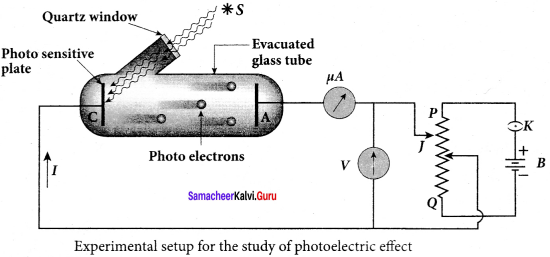3. The anode (positive electrode) A collects the electrons emitted from C. These electrodes are taken in an evacuated glass envelope with a quartz window that permits the passage of ultraviolet and visible light.

4. The necessary potential difference between C and A is provided by high tension battery B which is connected across a potential divider arrangement PQ through a key K. C is connected to the centre terminal while A to the sliding contact J of the potential divider.

5. The plate A can be maintained at a desired positive or negative potential with respect to C. To measure both positive and negative potential of A with respect to C, the voltmeter is designed to have its zero marking at the centre and is connected between A and C. The current is measured by a micro ammeter μA in series.

6. If there is no light falling on the cathode C, no photoelectrons are emitted and the microammeter reads zero. When ultraviolet or visible light is allowed to fall on C, the photoelectrons are liberated and are attracted towards anode.

7. As a result, the photoelectric current is setup in the circuit which is measured using micro ammeter.

8.  The variation of photocurrent with respect to-

1.  intensity of incident light
2. the potential difference between the electrodes
3. the nature of the material and
4. frequency of incident light can be studied with the help of this apparatus.

Question 2.
Write down the characteristics of photons.
Characteristics of photons:
According to particle nature of light, photons are the basic constituents of any radiation and possess the following characteristic properties:
(i) The photons of light of frequency v and wavelength λ will have energy, given by
E = hυ = $$\frac { hc }{ λ }$$.

(ii) The energy of a photon is determined by the frequency of the radiation and not by its intensity and the intensity has no relation with the energy of the individual photons in the beam.

(iii) The photons travel with the velocity of light and its momentum is given by p

(iv) Since photons are electrically neutral, they are unaffected by electric and magnetic fields.

(v) When a photon interacts with matter (photon-electron collision), the total energy, total linear momentum and angular momentum are conserved. Since photon may be absorbed or a new photon may be produced in such interactions, the number of photons may not be conserved

Question 3.
Briefly explain the nature of light, (wave-particle duality)
The nature of light: wave – particle duality
We have learnt that wave nature of light explains phenomena such as interference, diffraction and polarization. Certain phenomena like black body radiation, photoelectric effect can be explained by assigning particle nature to light. Therefore, both theories have enough experimental evidences.

In the past, many scientific theories have been either revised or discarded when they contradicted with new experimental results. Here, two different theories are needed to answer the question: what is nature of light?
It is therefore concluded that light possesses dual nature, that of both particle and wave. It behaves like a wave at some circumstances and it behaves like a particle at some other circumstances.

In other words, light behaves as a wave during its propagation and behaves as a particle during its interaction with matter. Both theories are necessary for complete description of physical phenomena. Hence, the wave nature and quantum nature complement each other.

Question 4.
Derive de-Broglie wave equation (wavelength) for a material particle.
De Broglie wave length:
The momentum of photon of frequency v is given by
p = $$\frac { hυ }{ c }$$ = $$\frac { h }{ λ }$$ since c = υλ
The wavelength of a photon in terms of its momentum is
λ = $$\frac { h }{ p }$$ …(1)
According to de Broglie, the above equation is completely a general one and this is applicable to material particles as well. Therefore, for a particle of mass m travelling with speed v , the wavelength is given by
λ = $$\frac { h }{ mv }$$ = $$\frac { h }{ p }$$ ….. (2)
This wavelength of the matter waves is known as de Broglie wavelength. This equation relates the wave character (the wave length λ) and the particle character (the momentum p) through Planck’s constant.

Question 5.
Explain the production of X-rays.
Production of x-rays:
X-rays are produced in x-ray tube which is essentially a discharge tube. A tungsten filament F is heated to incandescence by a battery. As a result, electrons are emitted from it by thermionic emission.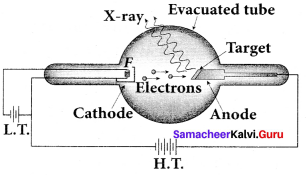The electrons are accelerated to high speeds by the voltage applied between the filament F and the anode. The target materials like tungsten, molybdenum are embedded in the face of the solid copper anode. The face of the target is inclined at an angle with respect to the electron beam so that x-rays can leave the tube through its side.

When high-speed electrons strike the target, they are decelerated suddenly and lose their kinetic energy. As a result, x-ray photons are produced. Since most of the kinetic energy of the bombarding electrons gets converted into heat, targets made of high-meltmg-point metals and a cooling system are usually employed.

Question 6.
Briefly explain the concept of continuous X-ray spectra.
Continuous x-ray spectra:
When a fast moving electron penetrates and approaches a target nucleus, the interaction between the electron and the nucleus either accelerates or decelerates it which results in a change of path of the electron. The radiation produced from such decelerating electron is called Bremsstrahlung or braking radiation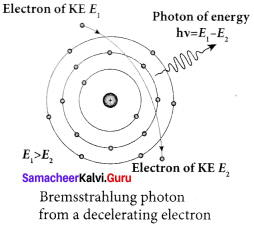The energy of the photon emitted is equal to the loss of kinetic energy of the electron. Since an electron may lose part or all of its energy to the photon, the photons are emitted with all possible energies (or frequencies). The continuous x-ray spectrum is due to such radiations.

When an electron gives up all its energy, then the photon is emitted with highest frequency υ0 (or lowest wavelength λ0 ). The initial kinetic energy of an electron is given by eV where V is the accelerating voltage. Therefore, we have
0 = eV (or) $$\frac { hc }{{ λ }_{0}}$$ = ev
λ0 = $$\frac { hc }{eV}$$
where λ0 is the cut-off wavelength. Substituting the known values in the above equation, we get
λ0 = $$\frac { 122400 }{V}$$ Å
The relation given by equation is known as the Duane – Hunt formula.
The value of λ0 depends only on the accelerating potential and is same for all targets. This is in good agreement with the experimental results. Thus, the production of continuous x-ray spectrum and the origin of cut – off wavelength can be explained on the basis of photon theory of radiation.

Question 7.
Write down the applications of X-rays.
Applications of x-rays:
X-rays are being used in many fields. Let us list a few of them.
1. Medical diagnosis:
X-rays can pass through flesh more easily than through bones. Thus an x-ray radiograph containing a deep shadow of the bones and a light shadow of the flesh may be obtained. X-ray radiographs are used to detect fractures, foreign bodies, diseased organs etc.

2. Medical therapy:
Since x-rays can kill diseased tissues, they are employed to cure skin diseases, malignant tumours etc.

3. Industry:
X-rays are used to check for flaws in welded joints, motor tyres, tennis balls and wood. At the custom post, they are used for detection of contraband goods.

4. Scientific research:
X-ray diffraction is important tool to study the structure of the crystalline materials – that is, the arrangement of atoms and molecules in crystals.

Samacheer Kalvi 12th Physics Dual Nature of Radiation and Matter Additional Numerical Problems

Question 1.
If a light of wavelength 4950 Å is viewed as a continuous flow of photons, what is the energy of each photon in eV? (Given h = 6.6 x 10-34 Js, c = 3 x 108 ms-1)
Solution:
Here λ = 4950 Å = 4950 x 10-10 m
Energy of each photon,
E = $$\frac { hc }{λ}$$ = $$\frac{6.6 \times 10^{-34} \times 3 \times 10^{8}}{4950 \times 10^{-10}}$$ = 4 x 10-19 J
= $$\frac{4 \times 10^{-19}}{1.6 \times 10^{-19}}$$eV
E = 2.5 eV

Question 2.
Monochromatic light of frequency 6 x 1014 Hz is produced by a laser. The power emitted is 2 x 10-3w.
(i) What is the energy of each photon in the light?
(ii) How many photons per second, on the average, are emitted by the source?
Solution:
(i) Energy of each photon,
E = hυ = 6.6 x 10-34 x 6 x 1014
E = 3.98 x 10-19J
(ii) If N is the number of photons emitted per second by the source, then
Power transmitted in the beam = N x energy of each photon
P = N
N = $$\frac { P }{ E }$$ = $$\frac{2 \times 10^{-3}}{3.98 \times 10^{-19}}$$
N = 5 x 1015 Photons per second.

Question 3.
Light of wavelength 5000 Å falls on a metal surface of work function 1.9 eV. Find:
(i) the energy of photons in eV
(ii) the K.E of photoelectrons and
(iii) the stopping potential.
Solution:
Here λ = 5000 Å = 5 x 10-7 m
W0 = 1.9 ev
(i) Energy of a photon,
E = $$\frac { hc }{λ}$$ = $$\frac{6.6 \times 10^{-34} \times 3 \times 10^{8}}{5 \times 10^{-7}}$$ J = $$\frac{6.6 \times 10^{-34} \times 3 \times 10^{8}}{5 \times 10^{-7} \times 1.6 \times 10^{-19}} e V$$ eV
E = 2.475 eV
(ii) K.E of a photoelectron,
K.E = hυ – W0 = 2.475 – 1.9 = 0.575 eV
(iii) Let V0 be the stopping potential. Then
eV0 = $$\frac { 1 }{ 2 }$$ mv2 = K.E of a photoelectron
V0 = $$\frac { 0.575 }{ e }$$ eV
V0 = 0.575 V

Question 4.
If photoelectrons are to be emitted from a potassium surface with a speed 6 x 106 ms-1, what frequency of radiation must be used? (Threshold frequency for potassium is 4.22 x 1014 Hz, h = 6.6 x 10-34 Js, me = 9.1 x 10-31 kg)
Solution:
Here, v = 6 x 106 ms-1
V0 = 4.22 x 1014 Hz
From Einstein’s photoelectric equation,
k.E = $$\frac { 1 }{ 2 }$$ mv2 = h (υ – υ0)
υ = $$\frac { 1 }{ 2 }$$ $$\frac {{ mv }^{2}}{ h }$$ + υ0
= $$\frac { 1 }{ 2 }$$ x $$\frac{9.1 \times 10^{-31}+\left(6 \times 10^{6}\right)^{2}}{6.6 \times 10^{-34}}$$ + 4. 22 x 10-14
= (2.48 x 1014) + (4. 22 x 1014)
υ = 6.7 x1014 Hz

Question 5.
The photoelectric cut-off voltage in a certain experiment 1.5 V. What is the maximum kinetic energy of photoelectrons emitted?
Solution:
Here V0 = 1.5 V
Kmax = eV0 = 1.5 eV
= 1.5 x 1.6 x 10-19 J
Kmax = 24 x 10-19 J

Question 6.
What is the (a) momentum, (b) speed, and (c) de-Broglie wavelength of an electron with kinetic energy of 120 eV.
Solution:
Kinetic energy, K.E = 120 eV = 120 x 1.6 x 10-19
K = K.E = 1.92 x 10-17 J
(a) Momentum of an electron, P = $$\sqrt { 2mK }$$
P = $$\sqrt{2 \times 9.1 \times 10^{-31} \times 1.92 \times 10^{-17}}$$
P = 5.91 x 10-24 kg ms-1
(b) Speed of an electron,
v = $$\frac { p }{ m }$$ = $$\frac{5.91 \times 10^{-24}}{9.1 \times 10^{-31}}$$ = 6.5 x 106 kg ms-1
(c) de-Broglie wavelength,
λ = $$\frac { h }{ p }$$ = $$\frac{6.6 \times 10^{-34}}{5.91 \times 10^{-24}}$$ = 1.117 x 10-10 = 0.112 x 10-9 m
λ = 0.112 nm

Question 7.
An electron and a photon each have a wavelength of 1 nm. Find, (a) their momenta (b) the energy of the photon, and (c) kinetic energy of electron.
Solution:
(a) Both electron and photon have same wavelength. so, they have same momentum also,
P = $$\frac { h }{ λ }$$ = $$\frac{6.6 \times 10^{-34}}{1 \times 10^{-9}}$$ = 6.6 x 10-25 kg ms-1
(b) Energy of a photon,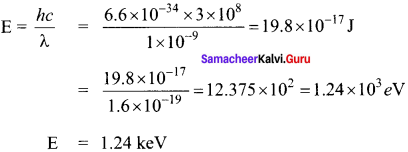(c) Kinetic energy of electron,
K = $$\frac {{ p }^{2}}{ 2m }$$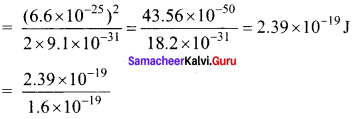K = 1.49 eV

Question 8.
Find the ratio of de-broglie wavelengths associated with two electron beams accelerated through 25 V and 36 V respectively.
Solution:
de-Broglie wavelength associate with potential difference λ ∝ $$\frac { 1 }{ √V }$$
$$\frac {{ λ }_{1}}{ { λ }_{2} }$$ = $$\sqrt { \frac { { V }_{ 2 } }{ { V }_{ 1 } } }$$ = $$\sqrt { \frac { 36 }{ 25 } }$$ = $$\frac { 6 }{ 5 }$$ ⇒ λ1 : λ2 = 6 : 5

Question 9.
A proton and an alpha particle, both initially at rest, are accelerated so as to have the same kinetic energy. What is the ratio of their de-Broglie wavelength?
Solution:
de-Broglie wavelength,
λ = $$\frac { h }{ p }$$ = $$\frac { h }{ \sqrt { 2mK } }$$
i.e.
λ ∝ $$\frac { 1 }{ √m}$$ [mα = 4mp]
$$\frac {{ λ }_{p}}{ { λ }_{α} }$$ = $$\sqrt { \frac { { m }_{ α } }{ { m }_{ p } } }$$ = $$\sqrt { \frac { { 4m }_{ p } }{ { m }_{ p } } }$$ = $$\sqrt { \frac { 4 }{ 1 } }$$ = $$\frac { 2 }{ 1 }$$
λp : λα = 2: 1

Question 10.
Light of two different frequencies whose photons have energies 1 eV and 2.5 eV respectively illuminate a metallic surface whose work function is 0.5 eV successively. Find the ratio of maximum speeds of emitted electrons.
Solution: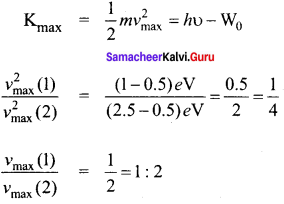We believe that the shared knowledge about Tamilnadu State Board Solutions for 12th Physics Chapter 7 Dual Nature of Radiation and Matter Questions and Answers learning resource will definitely guide you at the time of preparation. For more details visit Tamilnadu State Board Solutions for 12th Physics Solutions and for more information ask us in the below comments and we’ll revert back to you asap.